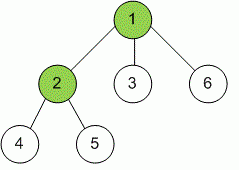Competitions

# Vertex Cover

You are given an unweighted, undirected tree. Write a program to find a vertex set of minimum size in this tree such that each edge has as least one of its end-points in that set.#### Input

The first line contains one integer n (0 < n100000) – number of nodes in the tree. Next n - 1 lines contain n - 1 edges of that tree. Each line contains a pair (u, v) means there is an edge between node u and node v (1u, vn).

#### Output

Print number of nodes in the satisfied vertex set on one line.

Time limit 1 second
Memory limit 128 MiB
Input example #1
6
1 2
1 3
2 4
2 5
1 6

Output example #1
2